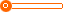# 彩妆加工 水晶3D四色眼影 立体缤纷眼影 炫彩裸色妆容必备 低价格

10000 个

10000 个

(发货期限：自买家付款之日起 天内发货)

• VIP指数:[第1年]
• 认证信息:
• 所在地区：广东
•• 详细说明
• 规格参数
• 联系方式
r r r r 颜色:多款供选r r r 色彩数:四色r r r r r 规格:正常规格r r r 品牌类型:精品国货r r r r r 净含量:可定制r r r rrr r r r r r r r r r r r r r r r r r  r r r

r
r

r

r
r
rr

r

r r

r

r
r

r

r 工厂加工样品图r

r

r
r
r
r
r
r
r
r
r
r

r

r
r

r r r r r r r  r r r r r r r r r r r r r r r r r r r r r r r r r r  r r r r r r r

r
r

r

r 本公司主营化妆品生产加工;彩妆生产加工销售;护肤品；膏霜、乳液、化妆水;洗涤；洗发水、沐浴露;眼影、腮红、口红、唇彩、眼线液、眼线膏等产品，欢迎广大新老客户前来选购！r

r

r
r

r

r 工厂样品间：r

r

r
r

r

r
r
r
r
r
r
r
r
r
r
r
r
r

r

r
r

r

r
r

r r r r r r r  r r r r r r r r r r r r r r r r r r r r r r r r r r  r r r r r r r

r
r

r

r  深圳市歌美姿化妆品有限公司创立于2009年，拥有正规化的生产基地，生产厂房3500平方米，专注于中高档化妆品品牌及半成品（OEM/ODM）的加工，拥有成熟的彩妆系列和护肤系列，是一家从事化妆品研发、策划、生产为一体的集团化妆品企业。
r    公司拥有高端的生产设备、先进的生产技术、准确的检测系统、优秀的生产团队；还有科学灵活的经营模式、合理完善的管理架构；更有着一股不满于现状，积极采纳国内外行业先进的生产技术和管理理念，勇于创新的精神。
r    公司以“追求美、缔造美、共享美”为企业核心，秉承着“质量第一、客户至上”的宗旨，与时俱进，积极创新，不断的自我完善、自我提升，以多样的产品，过硬的质量，合理的价格，优质的服务，与广大客户携手合作，共创未来。r

r

r
r

r r r r r r r  r r r r r r r r r r r r r r r r r r r r r r r r r r  r r r r r r r

r
r

r

r r

r

r
r

r r r r r r r  r r r r r r r r r r r r r r r r r"};

查看更多同类品牌产品
上一组 下一组

## 您可以通过以下类目找到类似信息：

免责声明：以上所展示的信息由会员自行提供，内容的真实性、准确性和合法性由发布会员负责。生活头条网对此不承担任何责任。

友情提醒：为规避购买风险，建议您在购买相关产品前务必确认供应商资质及产品质量！